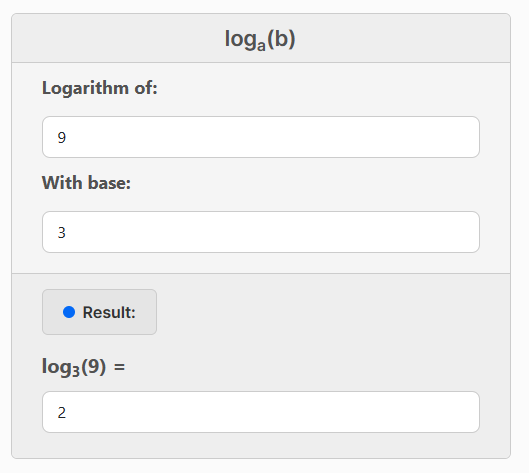# Logarithm Calculator

Calculate the logarithm of a given number with any base.

loga(b)
Result:
loga(b) =

A logarithm is a mathematical function that determines the exponent to which a specific base must be raised to obtain a given number.

For example, in the equation 102 = 100, the logarithm of 100 with base 10 is 2 because 10 raised to the power of 2 equals 100.

102 = 100
log10(100) = 2
More examples:
• 103 = 1000, so log10(1000) = 3
• 52 = 25, so log5(25) = 2
• 43 = 64, so log4(64) = 3
• 90 = 1, so log9(1) = 0

## How to use the Logarithm Calculator?

Using this calculator is very simple:

1. Fill in the "Logarith of" and "With base" fields.